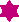# User Forum

Subject :IMO    Class : Class 6Which of the following statement(s) is/are true ?

(A) A parallelogram in which two adjacent angles are equal is a rectangle

(B) A quadrilateral in which both pairs of opposite angles are equal is parallelogram

(C) In a parallelogram the number of acute angles is zero (or) two

(D) All the above
how is (d) the correct answer